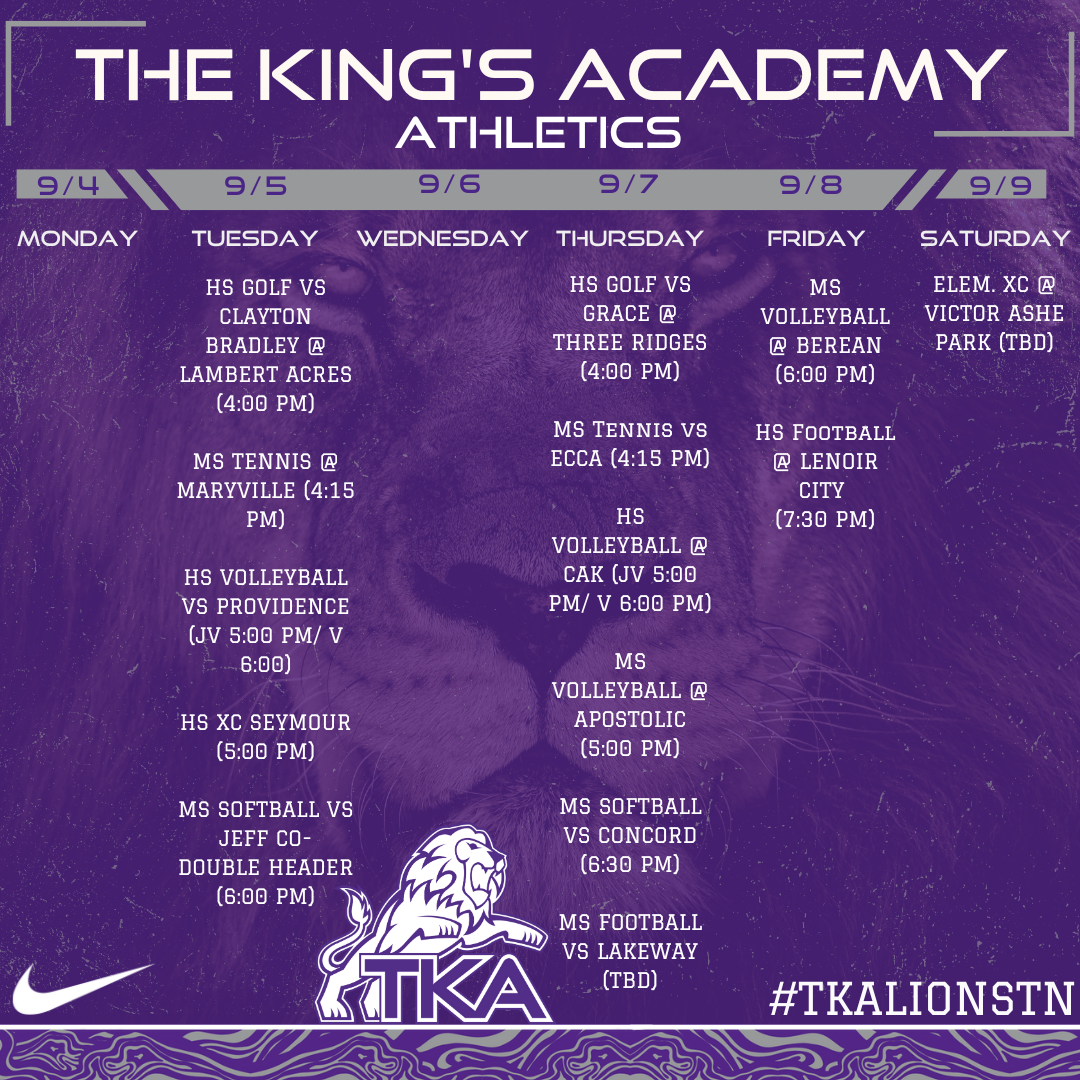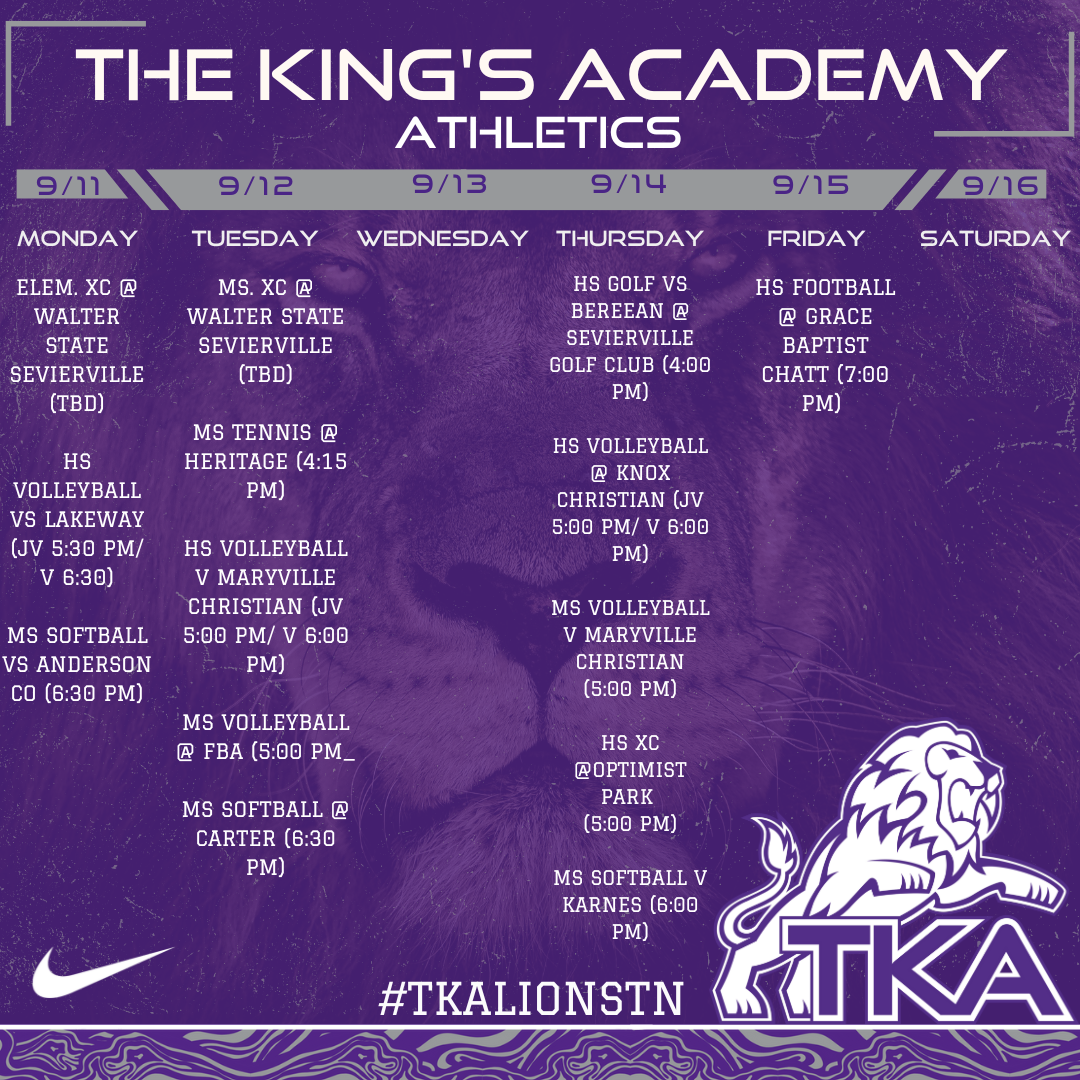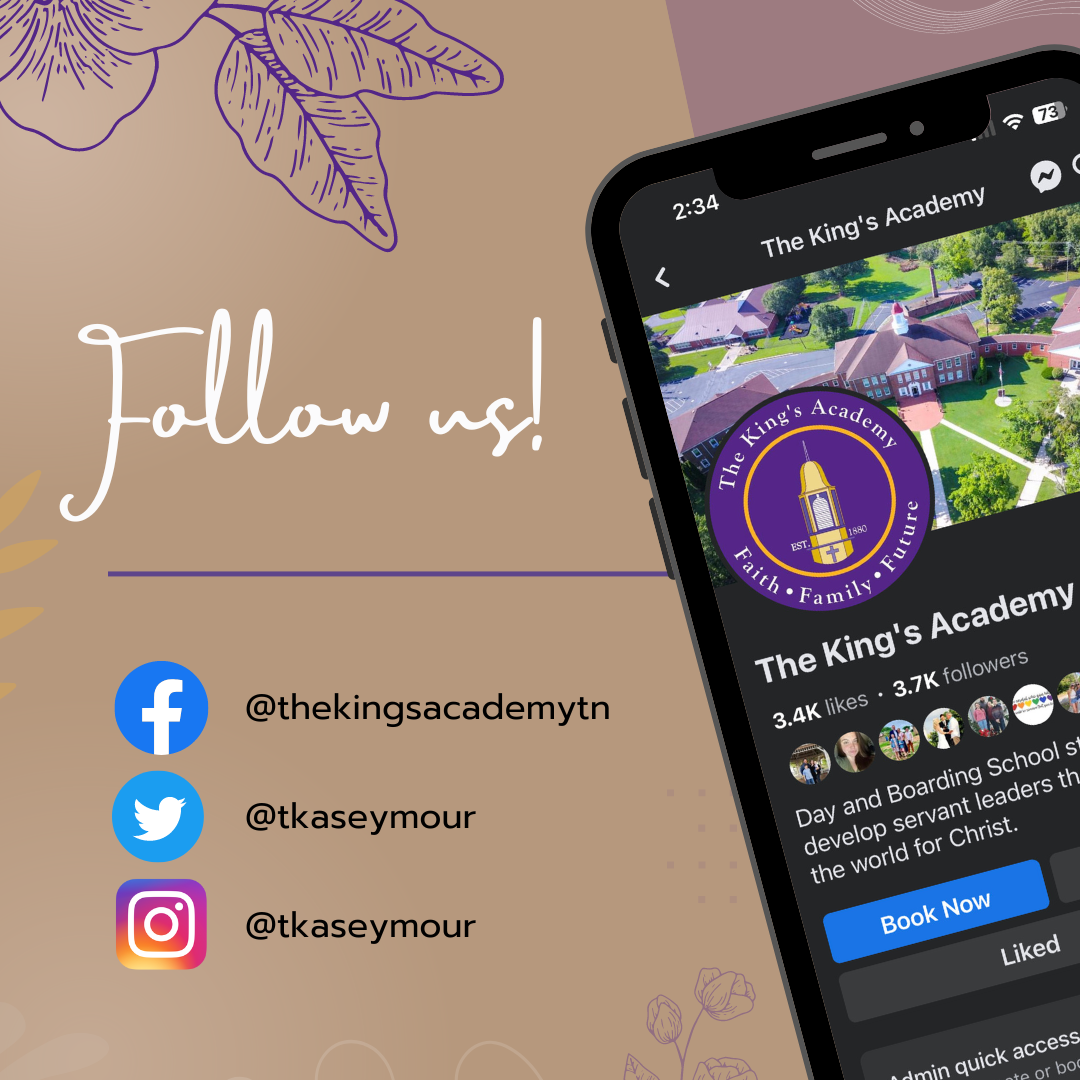## Lions Weekly: September 6, 2023# A Note From Mr. Sandefur

Lion Family,
As we step into another week here at The King's Academy, I'm reminded of the incredible privilege we have in nurturing the hearts and minds of our students. Our commitment to excellence extends beyond academics; it encompasses character development and spiritual growth. Our journey together, in pursuit of academic excellence and spiritual enrichment, is an ongoing testament to the incredible community that makes TKA so unique.

As we enter this week, let's focus on one of our core graduate profile attributes – being a "Critical Thinker." In Proverbs 14:15, it is written, "The simple believe anything, but the prudent give thought to their steps." Critical thinking is a hallmark of a well-rounded education, and it's central to our mission of nurturing students who can navigate the world with discernment and wisdom.

In a world filled with information, it's crucial that our students not only absorb knowledge but also learn how to evaluate it critically. This involves questioning, analyzing, and seeking a deeper understanding of the world around them. As parents and educators, we play a vital role in fostering this skill, guiding our students to engage thoughtfully with both the academic and spiritual aspects of their lives. Remember, our goal is not to tell them what to think but to teach them how to think – with reason, insight, and, most importantly, through the lens of their faith.

Thank you for entrusting us with your children's education and spiritual development. Together, we are nurturing the critical thinkers and faithful believers who will make a profound impact on the world. May God bless you and our TKA community abundantly in the coming week.

In Christ,

Jeremy Sandefur

 table div table+table+table+table div table{width:100%;padding:0}table div table+table+table+table div table img{width:96.23%;padding:0;float:none}table div table+table+table+table div table td{width:100%;padding:0 1.88% 18px}/* styles */table div table+table+table+table+table+table div table{width:100%;padding:0}table div table+table+table+table+table+table div table img{width:96.23%;padding:0;float:none}table div table+table+table+table+table+table div table td{width:100%;padding:0 1.88% 18px}/* styles */# Book Fair!

## 9/8-9/15

Join us for the exciting upcoming Book Fair from September 8th to September 15th! Get ready to dive into the world of captivating stories and knowledge. For more information, click the link below. Don't miss out on this literary adventure!

 table div table+table+table+table+table+table+table+table+table div table{width:100%;padding:0}table div table+table+table+table+table+table+table+table+table div table img{width:96.23%;padding:0;float:none}table div table+table+table+table+table+table+table+table+table div table td{width:100%;padding:0 1.88% 18px}/* styles */# Grandparents' Day

Don't forget, this Friday is a special day at The King's Academy as we celebrate Grandparents' Day! We invite all elementary grandparents and grandfriends to join us for a memorable morning filled with love and laughter. It's a fantastic opportunity to strengthen family bonds and create cherished memories. We look forward to welcoming you to our campus and celebrating this day together as a community. See you on Friday!

 table div table+table+table+table+table+table+table+table+table+table+table div table{width:100%;padding:0}table div table+table+table+table+table+table+table+table+table+table+table div table img{width:96.23%;padding:0;float:none}table div table+table+table+table+table+table+table+table+table+table+table div table td{width:100%;padding:0 1.88% 18px}/* styles */# TKA Junior League!

Is your 2nd - 5th grader interested in playing basketball for TKA's Jr. League teams this year? Please complete this brief 'TKA Jr. League Basketball Pre-Registration Form' that will assist us in determining interest and developing preliminary rosters. Once completed, our Junior League leadership team will evaluate the responses and reach out to you with additional information.

Jr. League is a competitive elementary basketball league which typically consists of 1-2 practices per week beginning in September. Games / tournaments begin in early November in gyms throughout Sevier County (TKA included) and last through early-February.

We look forward to hearing from you!

tkahoops@gmail.com

 table div table+table+table+table+table+table+table+table+table+table+table+table+table+table div table{width:100%;padding:0}table div table+table+table+table+table+table+table+table+table+table+table+table+table+table div table img{width:96.23%;padding:0;float:none}table div table+table+table+table+table+table+table+table+table+table+table+table+table+table div table td{width:100%;padding:0 1.88% 18px}/* styles */# Home Athletic Ticket Purchases

Get ready for an exciting season of TKA athletics this fall! We're thrilled to offer a convenient way to purchase your tickets for all our home events. The GoFan link below provides options for season tickets covering all our fall sports, as well as individual tickets for specific games. As part of our efforts to provide a safe and streamlined experience, we're aiming for cashless transactions at the gates. Please share these links with your families and friends to help ensure a smooth entry to our games. Remember, all TKA students get in for free. If you have any questions, don't hesitate to reach out.
-Coach Jones

 table div table+table+table+table+table+table+table+table+table+table+table+table+table+table+table+table+table div table{width:100%;padding:0}table div table+table+table+table+table+table+table+table+table+table+table+table+table+table+table+table+table div table img{width:96.23%;padding:0;float:none}table div table+table+table+table+table+table+table+table+table+table+table+table+table+table+table+table+table div table td{width:100%;padding:0 1.88% 18px}/* styles */# Legacy Matters: Conversations on Faith, Family, and Future

Don't miss out on the latest episode of our podcast, "Legacy Matters: Conversations on Faith, Family, and Future." It's a fantastic way to gain insights into our school's mission and values. Our next episode will be released next Tuesday, September 12th, and you won't want to miss the engaging discussions we have in store. Tune in for a deeper dive into our school's principles and hear from our dedicated team. Subscribe and stay connected with us as we explore important topics relevant to our school community.

 table div table+table+table+table+table+table+table+table+table+table+table+table+table+table+table+table+table+table+table+table+table+table div table{width:100%;padding:0}table div table+table+table+table+table+table+table+table+table+table+table+table+table+table+table+table+table+table+table+table+table+table div table img{width:96.23%;padding:0;float:none}table div table+table+table+table+table+table+table+table+table+table+table+table+table+table+table+table+table+table+table+table+table+table div table td{width:100%;padding:0 1.88% 18px}/* styles */# Book Fair Volunteers Needed!

The TKA Book Fair is just around the corner. Mrs. Durham is in need of volunteers to help set up, to help Little Lions shop, to help with the cash register, and to help take down. If you would be willing to help, please sign up using the link below. Volunteers are needed Thursday, September 7 - Friday, September 15.

The book fair will begin on Grandparents Day (September 8) and run through the following week (September 15). Look for more information about the fair in next week's email.

 table div table+table+table+table+table+table+table+table+table+table+table+table+table+table+table+table+table+table+table+table+table+table+table+table+table div table{width:100%;padding:0}table div table+table+table+table+table+table+table+table+table+table+table+table+table+table+table+table+table+table+table+table+table+table+table+table+table div table img{width:96.23%;padding:0;float:none}table div table+table+table+table+table+table+table+table+table+table+table+table+table+table+table+table+table+table+table+table+table+table+table+table+table div table td{width:100%;padding:0 1.88% 18px}/* styles */Calling all compassionate hearts! If you're interested in making a meaningful difference in the lives of our Residence Life students, we invite you to join our Residence Life Facebook Page. Whether you're a parent, staff member, or community member, your support matters. Stay updated on opportunities to mentor, provide encouragement, or contribute to their well-being. Together, we can create a warm and nurturing environment that helps our students thrive. Join us on this journey of impact and transformation!

 table div table+table+table+table+table+table+table+table+table+table+table+table+table+table+table+table+table+table+table+table+table+table+table+table+table+table+table+table div table{width:100%;padding:0}table div table+table+table+table+table+table+table+table+table+table+table+table+table+table+table+table+table+table+table+table+table+table+table+table+table+table+table+table div table img{width:96.23%;padding:0;float:none}table div table+table+table+table+table+table+table+table+table+table+table+table+table+table+table+table+table+table+table+table+table+table+table+table+table+table+table+table div table td{width:100%;padding:0 1.88% 18px}/* styles */## Homecoming Information!# TURN UP THE SPIRIT!

Get ready to ignite the school spirit as we announce this year's thrilling Homecoming theme: "Turn Up the Spirit!" Prepare for an unforgettable week of festivities, camaraderie, and celebration of our Lion spirit our Lion pride like never before.

 table div table+table+table+table+table+table+table+table+table+table+table+table+table+table+table+table+table+table+table+table+table+table+table+table+table+table+table+table+table+table+table+table div table{width:100%;padding:0}table div table+table+table+table+table+table+table+table+table+table+table+table+table+table+table+table+table+table+table+table+table+table+table+table+table+table+table+table+table+table+table+table div table img{width:96.23%;padding:0;float:none}table div table+table+table+table+table+table+table+table+table+table+table+table+table+table+table+table+table+table+table+table+table+table+table+table+table+table+table+table+table+table+table+table div table td{width:100%;padding:0 1.88% 18px}/* styles */# Now Hiring!

We have a new opportunity available in our Food Services Department at The King's Academy. We have both full-time and part-time positions, including part-time roles during evenings and weekends (Saturday and Sunday, 9 AM - 7 PM). If you're passionate about great food and service, we'd love to hear from you. Join us in providing top-notch dining experiences at TKA!

 table div table+table+table+table+table+table+table+table+table+table+table+table+table+table+table+table+table+table+table+table+table+table+table+table+table+table+table+table+table+table+table+table+table+table+table div table{width:100%;padding:0}table div table+table+table+table+table+table+table+table+table+table+table+table+table+table+table+table+table+table+table+table+table+table+table+table+table+table+table+table+table+table+table+table+table+table+table div table img{width:96.23%;padding:0;float:none}table div table+table+table+table+table+table+table+table+table+table+table+table+table+table+table+table+table+table+table+table+table+table+table+table+table+table+table+table+table+table+table+table+table+table+table div table td{width:100%;padding:0 1.88% 18px}/* styles */## Upcoming Sports Scheduletable div table+table+table+table+table+table+table+table+table+table+table+table+table+table+table+table+table+table+table+table+table+table+table+table+table+table+table+table+table+table+table+table+table+table+table+table+table+table+table div table{width:100%;padding:0}table div table+table+table+table+table+table+table+table+table+table+table+table+table+table+table+table+table+table+table+table+table+table+table+table+table+table+table+table+table+table+table+table+table+table+table+table+table+table+table div table img{width:96.23%;padding:0;float:none}table div table+table+table+table+table+table+table+table+table+table+table+table+table+table+table+table+table+table+table+table+table+table+table+table+table+table+table+table+table+table+table+table+table+table+table+table+table+table+table div table td{width:100%;padding:0 1.88% 18px}/* styles */table div table+table+table+table+table+table+table+table+table+table+table+table+table+table+table+table+table+table+table+table+table+table+table+table+table+table+table+table+table+table+table+table+table+table+table+table+table+table+table+table+table+table+table div table{width:100%;padding:0}table div table+table+table+table+table+table+table+table+table+table+table+table+table+table+table+table+table+table+table+table+table+table+table+table+table+table+table+table+table+table+table+table+table+table+table+table+table+table+table+table+table+table+table div table img{width:96.23%;padding:0;float:none}table div table+table+table+table+table+table+table+table+table+table+table+table+table+table+table+table+table+table+table+table+table+table+table+table+table+table+table+table+table+table+table+table+table+table+table+table+table+table+table+table+table+table+table div table td{width:100%;padding:0 1.88% 18px}/* styles */# Instructional Calendar

 table div table+table+table+table+table+table+table+table+table+table+table+table+table+table+table+table+table+table+table+table+table+table+table+table+table+table+table+table+table+table+table+table+table+table+table+table+table+table+table+table+table+table+table+table+table+table div table td,table.module-45{width:100%;padding:0}table div table+table+table+table+table+table+table+table+table+table+table+table+table+table+table+table+table+table+table+table+table+table+table+table+table+table+table+table+table+table+table+table+table+table+table+table+table+table+table+table+table+table+table+table+table+table div table{width:100%;float:none;margin-left:auto;margin-right:auto;padding:0}table div table+table+table+table+table+table+table+table+table+table+table+table+table+table+table+table+table+table+table+table+table+table+table+table+table+table+table+table+table+table+table+table+table+table+table+table+table+table+table+table+table+table+table+table+table+table div table a{border:0 none;text-decoration:none}table div table+table+table+table+table+table+table+table+table+table+table+table+table+table+table+table+table+table+table+table+table+table+table+table+table+table+table+table+table+table+table+table+table+table+table+table+table+table+table+table+table+table+table+table+table+table div table img{width:100%!important;border:0 none;text-decoration:none}/* styles */

# Dress Code

 table div table+table+table+table+table+table+table+table+table+table+table+table+table+table+table+table+table+table+table+table+table+table+table+table+table+table+table+table+table+table+table+table+table+table+table+table+table+table+table+table+table+table+table+table+table+table+table+table div table td,table.module-47{width:100%;padding:0}table div table+table+table+table+table+table+table+table+table+table+table+table+table+table+table+table+table+table+table+table+table+table+table+table+table+table+table+table+table+table+table+table+table+table+table+table+table+table+table+table+table+table+table+table+table+table+table+table div table{width:100%;float:none;margin-left:auto;margin-right:auto;padding:0}table div table+table+table+table+table+table+table+table+table+table+table+table+table+table+table+table+table+table+table+table+table+table+table+table+table+table+table+table+table+table+table+table+table+table+table+table+table+table+table+table+table+table+table+table+table+table+table+table div table a{border:0 none;text-decoration:none}table div table+table+table+table+table+table+table+table+table+table+table+table+table+table+table+table+table+table+table+table+table+table+table+table+table+table+table+table+table+table+table+table+table+table+table+table+table+table+table+table+table+table+table+table+table+table+table+table div table img{width:100%!important;border:0 none;text-decoration:none}/* styles */

## Dress Code Resources

 table div table+table+table+table+table+table+table+table+table+table+table+table+table+table+table+table+table+table+table+table+table+table+table+table+table+table+table+table+table+table+table+table+table+table+table+table+table+table+table+table+table+table+table+table+table+table+table+table+table+table+table div table{width:100%;padding:0}table div table+table+table+table+table+table+table+table+table+table+table+table+table+table+table+table+table+table+table+table+table+table+table+table+table+table+table+table+table+table+table+table+table+table+table+table+table+table+table+table+table+table+table+table+table+table+table+table+table+table+table div table img{width:96.23%;padding:0;float:none}table div table+table+table+table+table+table+table+table+table+table+table+table+table+table+table+table+table+table+table+table+table+table+table+table+table+table+table+table+table+table+table+table+table+table+table+table+table+table+table+table+table+table+table+table+table+table+table+table+table+table+table div table td{width:100%;padding:0 1.88% 18px}/* styles */